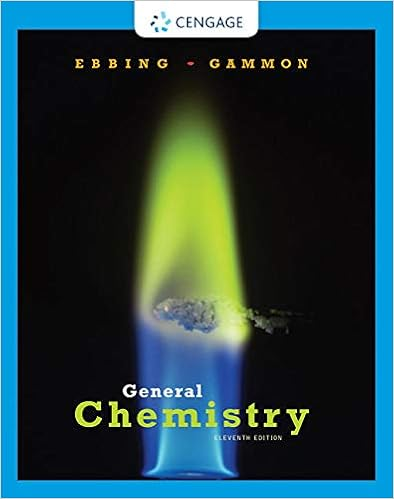# For the hydrogen atom schrodingers equation predicts

• Test Prep
• achin1004
• 4
• 93% (14) 13 out of 14 people found this document helpful

This preview shows page 1 - 4 out of 4 pages.

##### We have textbook solutions for you!
The document you are viewing contains questions related to this textbook.The document you are viewing contains questions related to this textbook.
Chapter 24 / Exercise 24.4
General Chemistry
Ebbing/GammonExpert Verified
5. For the hydrogen atom, Schrodingers’ Equation predicts that a. the 3s and 3p orbitals have the same energy b. the 2s has a larger energy than 2p c. the 2p has a larger energy than 2s d. the 1s and 2s have the same energy
##### We have textbook solutions for you!
The document you are viewing contains questions related to this textbook.The document you are viewing contains questions related to this textbook.
Chapter 24 / Exercise 24.4
General Chemistry
Ebbing/GammonExpert Verified
6. Which of the quantum numbers in NOT determined from the Schrodinger equation
n
l
s7. What is the energy for the 1st exited state of a harmonic oscillator
!
!
!
!
8. Which is NOT true, hydrogenase
9. If the spring constant is doubled for a given mass, the frequency a.increases by 2 b.decreases by 1/2 c.increases by 1.4 d.decreases by 1/1.4 ࠵?࠵?࠵?࠵?࠵?࠵?࠵?࠵?࠵?=࠵?࠵?2࠵?࠵?=1.41࠵?࠵?࠵?࠵?࠵?࠵?࠵?࠵?࠵?
10. Calculate the vibrational frequency for a proton, if k = 318 N/m
10
Part B. Open questions A. (10 points) Write (but do not solve) the average distance of an electron in a 2s orbital 1412࠵?࠵?!!(2࠵?࠵?!)࠵?!!/(!!!)!!!࠵?(4࠵?࠵?!࠵?࠵?)B. (10 points) Calculate the radial nodes for the2sorbital using the wavefunction ࠵?!"#࠵?=1412࠵?࠵?!
•••